Stochastic Curtailment

Lan, Simon, and Halperin (1982) introduce stochastic curtailment to stop a trial if, given current data, it is likely to predict the outcome of the trial with high probability. That is, a trial can be stopped to reject the null hypothesisif, given current data in the analyses, the conditional probability of rejectingunderat the end of the trial is greater than
, where the constantshould be between
andand values oforare recommended (Jennison and Turnbull 2000, p. 206). Similarly, a trial can be stopped to accept the null hypothesisif, given current data in the analyses, the conditional probability of rejectingunder the alternative hypothesis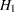at the end of the trial is less than.

The following two approaches for stochastic curtailment are available in the SEQTEST procedures: conditional power approach and predictive power approach. For each approach, the derived group sequential test is used as the reference test for rejection.

### Conditional Power Approach

In the SEQTEST procedure, the conditional power at an interim stageis the probability that the test statistic at the final stage (stage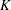) would exceed the rejection critical value (Cui, Hung, and Wang 1999, p. 854; Emerson, Kittelson, and Gillen 2005, p. 13). If there exist interim stages between theth stage and the final stage,, the conditional power is not the conditional probability to reject the null hypothesis. In this case, you can set the next stage as the final stage, and the conditional power is the conditional probability to reject.

The conditional distribution of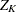given the observed statisticat theth stage and the hypothetical reference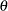is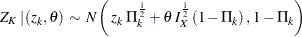where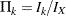is the fraction of information at theth stage.

The power for the upper alternative,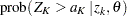, is then given bywhere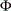is the cumulative distribution function of the standardized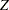statistic andis the upper critical value at the final stage.

Similarly, the power for the lower alternative,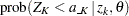, is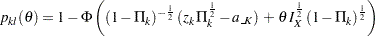whereis the lower critical value at the final stage.

A special case of the conditional power is the futility index (Ware, Muller, and Braunwald, 1985). It is one minus the conditional power under: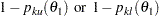That is, it is the probability of accepting the null hypothesis under the alternative hypothesis given current data. A high futility index indicates a small probability of success (rejecting) given the current data.

If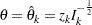, the maximum likelihood estimate at stage, the powers for the upper and lower alternatives can be simplified: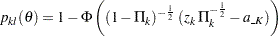### Predictive Power Approach

The conditional power depends on the specified reference, which might be supported by the current data (Jennison and Turnbull 2000, p. 210). An alternative is to use the predictive power (Herson 1979), which is a weighted average of the conditional power over values of. Without prior knowledge about, then with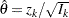, the maximum likelihood estimate at stage, the posterior distribution for(Jennison and Turnbull 2000, p. 211) is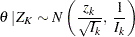Thus, the predictive power at stagefor the upper and lower alternatives can be derived as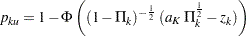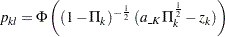whereandare the upper and lower critical values at the final stage.